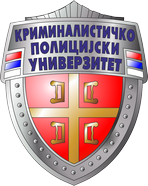THE UNIVERSITY OF CRIMINAL INVESTIGATION AND POLICE STUDIES

DOCTORAL STUDIES

FORENSIC ENGINEERING

Course:

# Mathematical Statistics with Reliability Theory

Course Code: 112.11

ECTS: 10

Course Status:

Elective

Number of Effective Classes (Per Week): 5

Theoretical Education:

Practical Training:

Research Study Project:

5

-

-

Prerequisite/s: None

Educational Objective: Getting acquainted with basic concepts and tools from probability theory, mathematical statistics, and reliability theory carrying out certain forensic analyzes. Understanding the statistical way of thinking and concluding, in general and specifically for forensic field. Training a student to choose the type of research data and mathematical-statistical procedures which they need for a specific task, as well as to actively make decisions based on statistical analysis and conclusions. Training a student for the application of mathematical-statistical methods in scientific research and in the adoption certain scientific and research conclusions in various scientific areas and in forensic scientific research.

Projected Outcome: The student is qualified to apply probability theory, mathematical statistics, and reliability theory in general and in forensics. He will be able to display and analyze data in various ways, apply statistical conclusions, identify and analyze specific distributions, make a sample and conduct sample analysis, calculate statistics on the basis of the sample. To select the type of research data and the mathematical-statistical procedures required for it a certain problem, as well as to actively make decisions based on statistical analysis and conclusions. Also, a student is qualified for the application of mathematical-statistical methods in scientific research and in the adoption of certain scientific-research conclusions in various scientific fields and in forensic scientific research.

Course Contents/Structure

Theoretical education: Basic concepts of probability theory. Random events, event algebras, probability definitions, conditional probability, formula of total probability, Bayes’ formula. Bernoulli's formula. Random variable. Two-dimensional random variable. Distribution of the random variable function. Basic distributions of discrete type. Basic distribution of continuous type. Laws of large numbers and central boundary theorem. Random processes and Marks chains. Sample, population, basic statistics and their distribution. Statistical models applied to problem solving predictions, classifications and groupings of high-dimensional data in the processes of in-depth analysis. Selected linear models, selected multivariate research techniques. Point and interval estimates of parameters. Testing hypothesis: parametric and non-parametric tests. Variance analysis. Regression and correlation. Planning a statistical experiment. Random numbers. Method Monte Carlo. Modeling of random variables. Basic concepts of reliability theory. Reliability active element to first failure. Reliability of the restored element. Reliability of the system.

Practical training: Solving tasks from theoretical education topics.

Teaching Methods: lecture, modified lecture, preparation of pre-exam research papers related to scientific research. Creation of graphic illustrations, expert and scientific presentations.

Assessment (Maximum Number of Points: 100)

Pre-Exam Obligations

No. of Points

Final Examination

No. of Points

Pre-exam research paper

30

Oral examination

70

Textbook/s

1. Hogg, R. V., McKean, J. W., Craig, A.T., “Introduction to Mathematical Statistics”, Harlow, Pearson Education, 2005

2. Larsen, R. J., Marx, M. L., ”An Introduction to Mathematical Statistics and Its Applications”, Upper Saddle River, N. J., Pearson / Prentice Hall, 2006

3. Merkle M., Vasić P., “Verovatnoća i statistika sa primenama i primerima”, (3. izd.) Beograd, Akademska misao, 2001

4. Petrović, Lj. “Teorijska statistika: teorija statističkog zaključivanja”, Beograd, Centar za izdavačku delatnost Ekonomskog fakulteta, 2006

5. Vukadinović, S. V., Popović, J. B., ”Matematička statistika Beograd”, Saobraćajni Fakultet Univerziteta, 2004